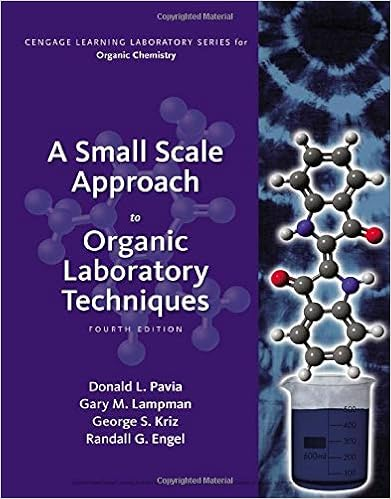# B c d e n mol a ans c page 69 chapter 5 gases and the

• Test Prep
• 19
• 92% (38) 35 out of 38 people found this document helpful

This preview shows page 4 - 7 out of 19 pages.

##### We have textbook solutions for you!
The document you are viewing contains questions related to this textbook.The document you are viewing contains questions related to this textbook.
Chapter T13 / Exercise 4
A Small Scale Approach to Organic Laboratory Techniques
Lampman/PaviaExpert Verified
bcden (mol)aAns: c
Page 69
##### We have textbook solutions for you!
The document you are viewing contains questions related to this textbook.The document you are viewing contains questions related to this textbook.
Chapter T13 / Exercise 4
A Small Scale Approach to Organic Laboratory Techniques
Lampman/PaviaExpert Verified
Chapter 5: Gases and the Kinetic-Molecular TheoryDifficulty: E22.A sample of an ideal gas has its volume doubled while its temperature remains constant.If the original pressure was 100 torr, what is the new pressure?
1000 torr
Difficulty: E23.A sample of the inert gas krypton has its pressure tripled while its temperature remainedconstant. If the original volume is 12 L, what is the final volume?
E) 48 L
Difficulty: E24.A weather balloon was initially at a pressure of 0.950 atm, and its volume was 35.0 L. The pressure decreased to 0.750 atm, without loss of gas or change in temperature. What was the change in the volume?
Difficulty: M25.A sample of nitrogen gas at 298 K and 745 torr has a volume of 37.42 L. What volume will it occupy if the pressure is increased to 894 torr at constant temperature?A) 22.3 L B) 31.2 L C) 44.9 L D) 112 L Ans: B
E) 380 L
Difficulty: E26.A sample of carbon dioxide gas at 125°C and 248 torr occupies a volume of 275 L. What will the gas pressure be if the volume is increased to 321 L at 125°C?
359 torr
Difficulty: E27.A sample of oxygen gas has its absolute temperature halved while the pressure of the gas remained constant. If the initial volume is 400 mL, what is the final volume?
800 mL
Difficulty: E28.A sample container of carbon monoxide occupies a volume of 435 mL at a pressure of 785 torr and a temperature of 298 K. What would its temperature be if the volume were changed to 265 mL at a pressure of 785 torr?
E) 538 K
Difficulty: MPage 70
Chapter 5: Gases and the Kinetic-Molecular Theory29.A 0.850-mole sample of nitrous oxide, a gas used as an anesthetic by dentists, has a volume of 20.46 L at 123°C and 1.35 atm. What would be its volume at 468°C and 1.35 atm?A) 5.38 L B) 10.9 L C) 19.0 L D) 38.3 L E) Ans: D
77.9 L
Difficulty: M30.A sample of ammonia gas at 65.5°C and 524 torr has a volume of 15.31 L. What is its volume when the temperature is –15.8°C and its pressure is 524 torr?
Difficulty: M31.A 500-mL sample of argon at 800 torr has its absolute temperature quadrupled. If the volume remains unchanged what is the new pressure?
3200 torr
Difficulty: E
•••